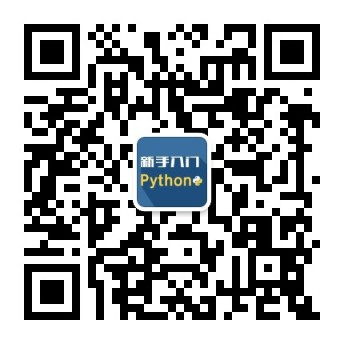2020
02-10

# Yii2中datetime类的使用

`\$datetime = new DateTime;`

`\$datetime = new \DateTime;`

```\$datetime = new \DateTime;
print_r(\$datetime->format('Y-m-d H:i:s'));```

```\$datetime = new \DateTime('2016-06-13');
print_r(\$datetime);```

```\$datetime = \DateTime::createFromFormat('Ymd', '20160618');
print_r(\$datetime->format('Y-m-d'));
```

```//方法1(php5.2)：
\$datetime = new \DateTime();
echo \$datetime->format('U');exit;

//方法2(php5.3)推荐
\$datetime = new \DateTime();
echo \$datetime->getTimestamp();exit;```

```\$datetime = new \DateTime();
\$datetime->setTimestamp(1465783744);
echo \$datetime->format('Y-m-d H:i:s');```

```
\$datetime1 = new \DateTime('2016-01-01 10:11:18');
\$datetime2 = new \DateTime('2017-05-11 22:21:21');
\$interval = \$datetime1->diff(\$datetime2);
print_r(\$interval->format('%Y'));```

//%表示使用格式化，R表示是大于这个日期(+)，还是小于这个日期（-）,
//a表示大于或小于多少天，时分秒正常使用y,m,d,h,i,s

DateInterval构造函数的参数是一个表示时间间隔约定的字符串，这个时间间隔约定以字母P开头，后面跟着一个整数，最后是一个周期标识符，限定前面的整数。有效周期标识符如下： Y（年） M（月） D（日） W（周） H（时） M（分） S（秒） 间隔约定中既可以有时间也可以有日期，如果有时间需要在日期和时间之间加上字母T，例如，间隔约定P2D表示间隔两天，间隔约定P2DT5H2M表示间隔两天五小时两分钟。

```
\$datetime = new \DateTime();
\$interval = new \DateInterval('P2DT5H');
//或者使用createFromDateString方法
//\$interval = \DateInterval::createFromDateString('1 month');
//修改DateTime实例
echo \$datetime->format('Y-m-d H:i:s');```

```
\$datetime = new \DateTime();
\$interval = new \DateInterval('P2DT5H');
\$datetime->sub(\$interval);
echo \$datetime->format('Y-m-d H:i:s');
//ps:有个modify方法，这个方法是减去30，并不是像前推1天，输出的还是12月
\$datetime = new \DateTime('2014/12/31');
\$datetime->modify( '-1 month' );
print_r(\$datetime);exit;
```

```\$datetime = new \DateTime();
\$datetime->setDate(2015, 2, 28);
echo \$datetime->format('Y-m-d');exit;```

```\$datetime = new \DateTime();
\$datetime->setTime(20, 20, 24);
echo \$datetime->format('Y-m-d H:i:s');exit;```

```\$timezone = new \DateTimeZone('Asia/Calcutta');
\$datetime = new \DateTime();
\$datetime->setTimezone(\$timezone);
print_r(\$datetime->format('Y-m-d H:i:s'));exit;```

```\$date = new \DateTime(null, new DateTimeZone('Asia/Shanghai'));
\$tz = \$date->getTimezone();
echo \$tz->getName();```

```\$dateTimeZoneTaipei = new \DateTimeZone("Asia/Taipei");
\$dateTimeZoneJapan = new \DateTimeZone("Asia/Tokyo");
\$dateTimeTaipei = new \DateTime("now", \$dateTimeZoneTaipei);
\$dateTimeJapan = new \DateTime("now", \$dateTimeZoneJapan);
\$timeOffset = \$dateTimeZoneJapan->getOffset(\$dateTimeTaipei);
print_r(\$timeOffset);exit;```

```\$interval = new \DateInterval('P2Y4DT6H8M');
echo \$interval->format('%d days');```

DatePeriod类的构造方法接受三个参数而且都必须提供 一个DateTime实例，表示迭代开始的日期和时间 一个DateInterval实例，表示下一个日期和时间的间隔 一个整数，表示迭代的总次数 第四个参数是可选的，用于显式指定周期的结束日期和时间，如果迭代时想要排除开始日期和时间，可以把构造方法的最后一个参数设为DatePeriod::EXCLUDE_START_DATE常量：

```\$datetime = new \DateTime();
\$interval = \DateInterval::createFromDateString('-1 day');
\$period = new \DatePeriod(\$datetime, \$interval, 3);
foreach (\$period as \$date) {
echo \$date->format('Y-m-d'), PHP_EOL;
}```